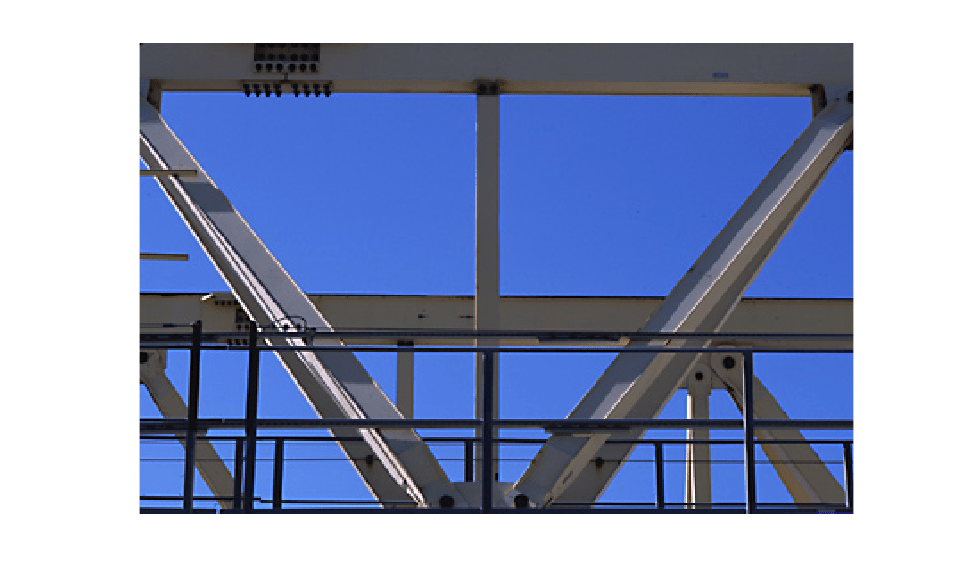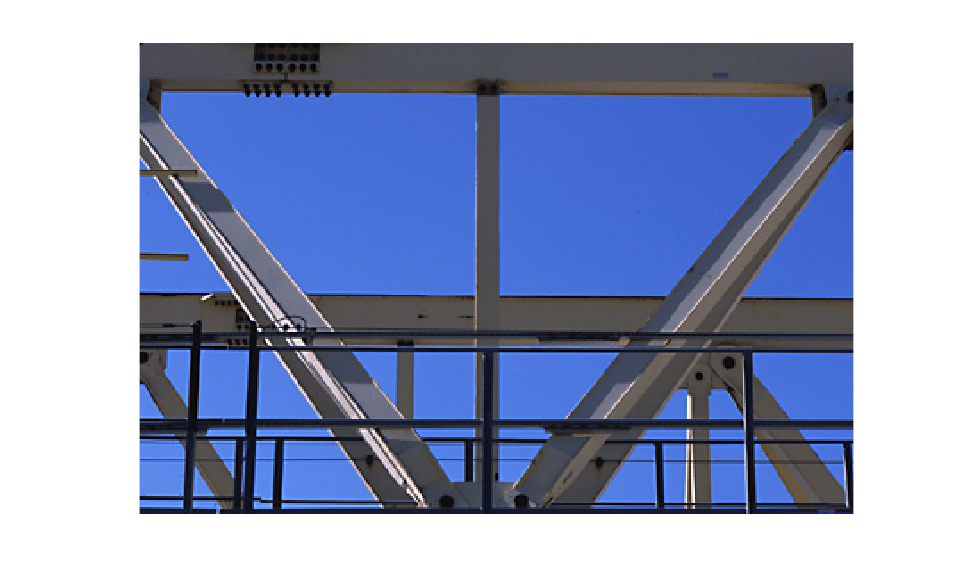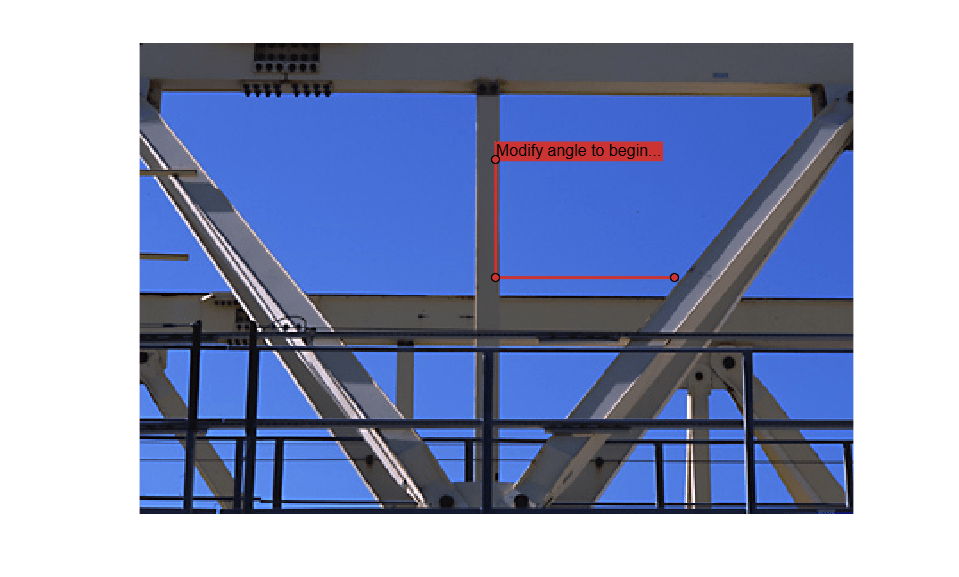Main Content

# Use Polyline to Create Angle Measurement Tool

This example shows how to create an interactive tool that displays the angle between three vertices in a polyline ROI.

You can change the angle by clicking and dragging the polyline vertices. When the ROI moves, it broadcasts an event named MovingROI. By adding a listener for that event and a callback function that executes when the event occurs, the tool can measure and display changes to the angle in real time.

### Display Image and Polyline ROI

Read and display an image.

```im = imread('gantrycrane.png'); imshow(im) ```Get the size of the image.

```[y,x,~] = size(im); ```

Get the coordinates of the center of the image. The example places the vertex of the angle measurement tool at the center of the image.

```midy = ceil(y/2); midx = ceil(x/2); ```

Specify the coordinates of the first point in the polyline ROI. This example places the first point in the polyline directly above the image center.

```firstx = midx; firsty = midy - ceil(y/4); ```

Specify the coordinates of the third point in the polyline ROI. This example places the third point in the polyline directly to the right of the image center.

```lastx = midx + ceil(x/4); lasty = midy; ```

Create an empty context menu to replace the default menu.

```c = uicontextmenu; ```Draw the polyline in red over the image. Specify the coordinates of the three vertices, and add a label with instructions to interact with the polyline.

```h = drawpolyline("Parent",gca, ... "Position",[firstx,firsty;midx,midy;lastx,lasty], ... "Label","Modify angle to begin...", ... "Color",[0.8,0.2,0.2], ... "UIContextMenu",c); ```Add a listener that listens for movement of the ROI. When the listener detects movement, it calls the custom callback function `updateAngle`. This custom function is defined in the section "Update Angle Label Using Callback Function".

```addlistener(h,'MovingROI',@(src,evt) updateAngle(src,evt)); ```

Polyline ROIs also support interactive addition and deletion of vertices. However, an angle measurement tool requires exactly three vertices at any time, so the addition and deletion of vertices are undesirable interactions with the ROI. Add listeners that listen for the addition or deletion of vertices. When you attempt to change the number of vertices, the appropriate listener calls a custom callback function to suppress the change. These custom functions, `storePositionInUserData` and `recallPositionInUserData`, are defined in the section "Prevent Addition or Deletion of Vertices Using Callback Functions".

```addlistener(h,'AddingVertex',@(src,evt) storePositionInUserData(src,evt)); addlistener(h,'VertexAdded',@(src,evt) recallPositionInUserData(src,evt)); addlistener(h,'DeletingVertex',@(src,evt) storePositionInUserData(src,evt)); addlistener(h,'VertexDeleted',@(src,evt) recallPositionInUserData(src,evt)); ```

### Update Angle Label Using Callback Function

Define a callback function that executes as the polyline ROI moves. This function retrieves the current position of the three vertices, calculates the angle in degrees between the vertices, and updates the label to display the current rotation angle.

```function updateAngle(src,evt) % Get the current position p = evt.CurrentPosition; % Find the angle v1 = [p(1,1)-p(2,1), p(1,2)-p(2,2)]; v2 = [p(3,1)-p(2,1), p(3,2)-p(2,2)]; theta = acos(dot(v1,v2)/(norm(v1)*norm(v2))); % Convert the angle to degrees angleDegrees = (theta * (180/pi)); % Update the label to display the angle src.Label = sprintf('(%1.0f) degrees',angleDegrees); end ```

### Prevent Addition or Deletion of Vertices Using Callback Functions

Define a callback function that executes when the listeners detect the 'AddingVertex' or 'DeletingVertex' events. These events occur immediately before the vertex of interest is added to or deleted from the polyline. Store the current three polyline vertices in the `UserData` property.

```function storePositionInUserData(src,~) src.UserData = src.Position; end ```

Define a callback function that executes when the listeners detect the 'VertexAdded' or 'VertexDeleted' events. These events occur immediately after the vertex of interest is added to or deleted from the polyline. Restore the stored set of three polyline vertices in the `UserData` property.

```function recallPositionInUserData(src,~) src.Position = src.UserData; end ```

Download ebook